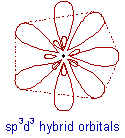# VALENCE BOND THEORY (VBT) & HYBRIDIZATION

The valence bond theory was proposed by Heitler and London to explain the formation of covalent bond quantitatively using quantum mechanics. Later on, Linus Pauling improved this theory by introducing the concept of hybridization.

The main postulates of this theory are as follows:

* A covalent bond is formed by the overlapping of two half filled valence atomic orbitals of two different atoms.

* The electrons in the overlapping orbitals get paired and confined between the nuclei of two atoms.

* The electron density between two bonded atoms increases due to overlapping. This confers stability to the molecule.

* Greater the extent of overlapping, stronger is the bond formed.

* The direction of the covalent bond is along the region of overlapping of the atomic orbitals i.e., covalent bond is directional.

* There are two types of covalent bonds based on the pattern of overlapping as follows:

(i) σ-bond: The covalent bond formed due to overlapping of atomic orbital along the inter nucleus axis is called σ-bond. It is a stronger bond and cylindrically symmetrical.

Depending on the types of orbitals overlapping, the σ-bond is divided into following types:

σs-s bond:σp-p bond: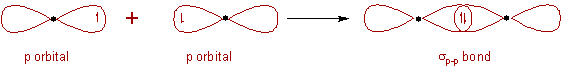σs-p bond:(ii) π-bond: The covalent bond formed by sidewise overlapping of atomic orbitals is called π- bond. In this bond, the electron density is present above and below the inter nuclear axis. It is relatively a weaker bond since the electrons are not strongly attracted by the nuclei of bonding atoms.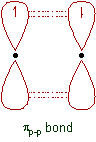Note: The 's' orbitals can only form σ-bonds, whereas the p, d & f orbitals can form both σ and π-bonds.

## BONDING IN MOLECULES EXPLAINED BY VALENCE BOND THEORY

1) H2 molecule:

* The electronic configuration of hydrogen atom in the ground state is 1s1

* In the formation of hydrogen molecule, two half filled 1s orbitals of hydrogen atoms overlap along the inter-nuclear axis and thus by forming a σs-s bond.2) Cl2 molecule:

* The electronic configuration of Cl atom in the ground state is [Ne]3s2 3px2 3py2 3pz1

* The two half filled 3pz atomic orbitals of two chlorine atoms overlap along the inter-nuclear axis and thus by forming a σp-p bond.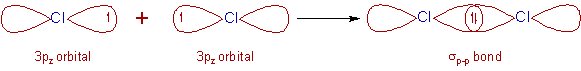3) HCl molecule:

* In the ground state, the electronic configuration of hydrogen atom is 1s1

* And the ground state electronic configuration of Cl atom is [Ne]3s2 3px2 3py2 3pz1

* The half filled 1s orbital of hydrogen overlap with the half filled 3pz atomic orbital of chlorine atom along the inter-nuclear axis to form a σs-p bond.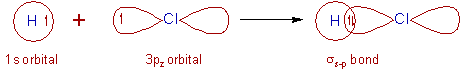4) O2 molecule:

* The electronic configuration of O in the ground state is [He] 2s2 2px2 2py1 2pz1.

* The half filled 2py orbitals of two oxygen atoms overlap along the inter-nuclear axis and form σp-p bond.

* The remaining half filled 2pz orbitals overlap laterally to form a πp-p bond.

* Thus a double bond (one σp-p and one πp-p) is formed between two oxygen atoms.5) N2 molecule:

* The ground state electronic configuration of N is [He] 2s2 2px1 2py1 2pz1.

* A σp-p bond is formed between two nitrogen atoms due to overlapping of half filled 2px atomic orbitals along the inter-nuclear axis.

* The remaining half filled 2py and 2pz orbitals form two πp-p bonds due to lateral overlapping. Thus a triple bond (one and two) is formed between two nitrogen atoms.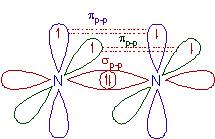### NEED FOR MODIFICATION OF VBT

However the old version of valence bond theory is limited to diatomic molecules only. It could not explain the structures and bond angles of molecules with more than three atoms.

E.g. It could not explain the structures and bond angles of H2O, NH3 etc.,

However, in order to explain the structures and bond angles of molecules, Linus Pauling modified the valence bond theory using hybridization concept.

## HYBRIDIZATION

### What is hybridization?

The intermixing of two or more pure atomic orbitals of an atom with almost same energy to give same number of identical and degenerate new type of orbitals is known as hybridization.

The new orbitals formed are also known as hybrid orbitals.

### What is intermixing?

The intermixing or hybridization of atomic orbitals is a mathematical concept based on quantum mechanics. During this process, the wavefunctions, Ψ  of atomic orbitals of same atom are combined to give new wavefunctions corresponding to hybrid orbitals.

### What are the requirements for atomic orbitals to undergo hybridization?

* The atomic orbitals of same atom with almost same energy can only participate in the hybridization.

* The full filled or half filled or even empty orbitals can undergo hybridization provided they have almost equal energy.

### Do the orbitals of different atoms undergo hybridization?

No! The hybridization is the mixing of orbitals of same atom only. The combination of orbitals belonging to different atoms is called bonding.

### What are hybrid orbitals? And what are its characteristics?

* The new orbitals that are formed due to intermixing of atomic orbitals are also known as hybrid orbitals, which have mixed characteristics of atomic orbitals.

* The shapes of hybrid orbitals are  identical. Usually they have one big lobe associated with a small lobe on the other side.

* The hybrid orbitals are degenerate i.e., they are associated with same energy.

### How many hybrid orbitals are formed?

* The number of hybrid orbitals formed is equal to the number of pure atomic orbitals undergoing hybridization.

E.g. If three atomic orbitals intermix with each other, the number of hybrid orbitals formed will be equal to 3.

### How do the electrons are going to be filled in the hybrid orbitals?

* The hybrid orbitals are filled with those electrons which were present in the pure atomic orbitals forming them.

* The filling up of electrons in them follows Pauli's exclusion principle and Hund's rule.

### What is the use of hybrid orbitals?

* The hybrid orbitals participate in the σ bond formation with other atoms.

### Why atomic orbitals in a given atom undergo hybridization?

* The hybrid orbitals are oriented in space so as to minimize repulsions between them. This explains why the atomic orbitals undergo hybridization before bond formation.

The reason for hybridization is to minimize the repulsions between the bonds that are going to be formed by the atoms by using hybrid orbitals.

Remember that the hybridization is the process that occurs before bond formation.

And finally:

* The bond angles in the molecule are equal to or almost equal to the angles between the hybrid orbitals forming the σ bonds. The shape of the molecule is determined by the type of hybridization, number of bonds formed by them and the number of lone pairs.

## TYPES OF HYBRIDIZATION

During hybridization, the atomic orbitals with different characteristics are mixed with each other. Hence there is no meaning of hybridization between same type of orbitals i.e., mixing of  two 's' orbitals or two 'p' orbitals is not called hybridization.

However orbital of 's' type can can mix with the orbitals of 'p' type or of 'd' type. Based on the type and number of orbitals, the hybridization can be subdivided into following types.

Note: Keep in mind that - only the orbitals of nearer energy values can participate in the hybridization.

## sp HYBRIDIZATION

What is sp hybridization?

* Intermixing of one 's' and one 'p' orbitals of almost equal energy to give two identical and degenerate hybrid orbitals is called 'sp' hybridization.

* These sp-hybrid orbitals are arranged linearly at by making 180o of angle.

* They possess 50% 's' and 50% 'p' character.## sp2 HYBRIDIZATION

What is sp2 hybridization?

* Intermixing of one 's' and two 'p' orbitals of almost equal energy to give three identical and degenerate hybrid orbitals is known as sp2 hybridization.

* The three sp2 hybrid orbitals are oriented in trigonal planar symmetry at angles of 120o to each other.

* The sp2 hybrid orbitals have 33.3% 's' character and 66.6% 'p' character.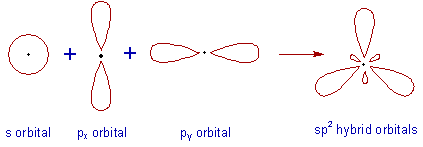## sp3 HYBRIDIZATION

What is sp3 hybridization?

* In sp3 hybridization, one 's' and three 'p' orbitals of almost equal energy intermix to give four identical and degenerate hybrid orbitals.

* These four sp3 hybrid orbitals are oriented in tetrahedral symmetry with 109o28' angle with each other.

* The sp3 hybrid orbitals have 25% ‘s’ character  and 75% 'p' character.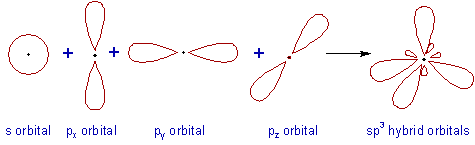## sp3d HYBRIDIZATION

What is sp3d hybridization?

* In sp3d hybridization, one 's', three 'p' and one 'd' orbitals  of almost equal energy intermix to give five identical and degenerate hybrid orbitals, which are arranged in trigonal bipyramidal symmetry.

Among them, three are arranged in trigonal plane and the remaining two orbitals are present above and below the trigonal plane at right angles.

* The sp3d hybrid orbitals have 20% 's', 60% 'p' and 20% 'd' characters.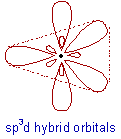## sp3d2 HYBRIDIZATION

What is sp3d2 hybridization?

* Intermixing of one 's', three 'p' and two 'd' orbitals of almost same energy by giving six identical and degenerate hybrid orbitals is called sp3d2 hybridization.

* These six sp3d2 orbitals are arranged in octahedral symmetry by making 90o angles to each other. This arrangement can be visualized as four orbitals arranged in a square plane and the remaining two are oriented above and below this plane perpendicularly.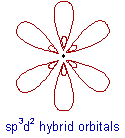## sp3d3 HYBRIDIZATION

What is sp3d3 hybridization?

* In sp3d3 hybridization, one 's', three 'p' and three 'd' orbitals of almost same energy intermix to give seven sp3d3 hybrid orbitals, which are oriented in pentagonal bipyramidal symmetry.

* Five among the sp3d3 orbitals are arranged in a pentagonal plane by making 72o of angles. The remaining are arranged perpendicularly above and below this pentagonal plane.